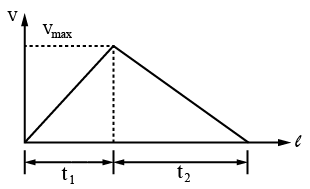# ISRO Scientist or Engineer Mechanical 2010

Instructions

For the following questions answer them individually

Question 11

# Choose the correct figure representing gas to gas heat transfer in parallel flow heat exchanger?Question 12

# The value of Prandtl number for air is aboutQuestion 13

# 4 body cools from $$90^\circ C to 80^\circ C$$ in 5 minutes. Under the same external conditions to cool from $$80^\circ C to 70^\circ C$$ the body will takeQuestion 14

# When the temperature of a solid surface changes from $$227^\circ C to 1227^\circ C$$ its total emissive power changes from $$E_1 to E_2.$$ The ratio $$\left(\frac {E_2} {E_1}\right)$$ will beQuestion 15

# Characteristic gas constant of a gas is equal toQuestion 16

# The matrix $$A = \begin{bmatrix}-4 & -3 & -3 \\1 & 0 & 1 \\4 & 4 & x \end{bmatrix}$$ is its own adjoint. The value of x will beQuestion 17

# The temperature of sun can be measured using aQuestion 18

# A car moving with uniform acceleration covers 450 m in a 5 second interval, and covers 700 m in the next 5 second interval. The acceleration of the car isQuestion 19

# A particle starts from rest with a constant acceleration $$\alpha m/sec^2$$ and after some time it decelerates at a uniform rate of $$\beta 8 m/sec^2$$ till it comes to rest. If the total time taken between two rests positions is t, the maximum velocity acquired by the particle would beQuestion 20

# Two metallic blocks having masses in the ratio 2: 3 are made to slide down a friction less inclined plane starting initially from rest position. When these blocks reach the bottom of the inclined plane, they will have their kinetic energies in the ratioOR